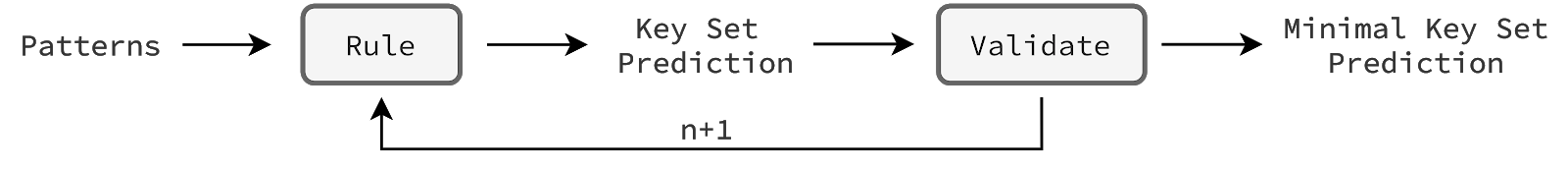12.
Inferring from Explanations: Complexity of Model Reasoning

#### Signing up to our newsletter allows you to read all our ebooks.

Do You Trust in Aspect-Based Sentiment Analysis?
12

# Inferring from Explanations: Complexity of Model Reasoning

Building better pattern recognizers is not the sole goal (at least, not in this article). The aim is to benefit from a recognizer so to be able to discover insights about a model (and a dataset), and use them to make improvements. For instance, the most attractive information we can infer from explanations is the complexity of model reasoning. In this section, we investigate whether a single token usually triggers off a model or a model rather uses more sophisticated structures. The analysis may quickly reveal any alarming model behaviors because it is rather suspicious if a single token stands behind a decision of a neural network. Even though it may be a valuable study on its own, the key concept of this section is to give an example of how to analyse error-prone explanations as a whole,  as opposed to reviewing them individually, something which might be misleading.

Assuming (roughly) that more complex structures engage more tokens (e.g. more relationships potentially), we can approximate the complexity of model reasoning by the number of tokens crucial to making a decision. We assume that crucial tokens are the minimal key set of tokens,  the minimal set of tokens that masked (altogether) cause a change in the model’s prediction. As a result, we can estimate the complexity using key sets that implicitly provide a pattern recognizer.

In this analysis, we benefit from the rule used in the last two tests that predicts (based on patterns) a key set of a given size n. To make this exemplary study clear, we want to be sure that analysing key sets is valid (causes a decision change). Therefore, we have introduced a simple policy that includes a validation. Namely, starting with the n=1 we iterate through key set predictions assuming that the first valid prediction is minimal. We validate a prediction by calling a model with the masked tokens (that belong to this set) expecting a decision change  (implementation is here).The chart below (on the left) presents a summary of model reasoning in terms of complexity. As we have said, the complexity is approximated by the number of masked tokens needed to change the model’s decision. We infer them from patterns using a plain rule and policy. The plot presents model reasoning on restaurant test predicted-negative examples. Because the dataset is unbalanced (positive examples dominate),  the model tends to classify masked examples as positive rather than neutral. This clearly demonstrates the confusion matrix on the right that keeps the original sentiment predictions in rows,  and the sentiment predictions after masking in columns. Look at the second row of predicted negative examples. Many examples after masking (either one, two or three masked tokens) change the sentiment to positive. We have investigated them and other positive predictions, and it turns out that almost all the fully-masked examples remain positive. Therefore, the analysis of predicted-negative examples is more informative because the predictions of positive sentiment would appear in the last column obscuring the overall picture. The problem occurs in both laptop and restaurant dataset domains (other plots are here).The decision in around 74% of cases is based on simple patterns wherein one or two masked tokens are enough to change a model’s prediction. The basic pattern recognizer provides a rough understanding but it is far from being perfect. The last column shows that in 47% of cases it cannot find (misclassified) a valid key token set. Note that the ground truth is helpful but not crucial. A more precise recognizer would try to push the predicted distribution towards the left-hand side, therefore, without ground truth, we can still reveal valuable insights about model reasoning.The analysis is done outside the pipeline (offline), to keep it clear. However, it can be easily adjusted to online monitoring of model behaviors. A key change concerns the number of additional calls to a model (preferably no calls). Instead of using a plain rule and policy, one can build another model that – based on patterns (or internal model states) – predicts whether an example has e.g. a key token or a key set of four tokens, without any extra calls to a model for verifications. As a result, the monitoring does not interfere with the model inference efficiency. The essence of this section is that we are able to track model behaviors e.g. complexity, and react if something goes wrong (or just changes rapidly).

PREVIOUS
Chapter
11
NEXT
Chapter
13